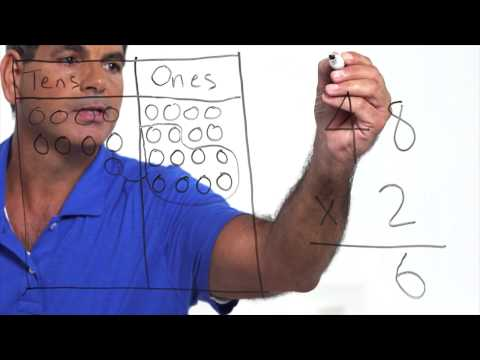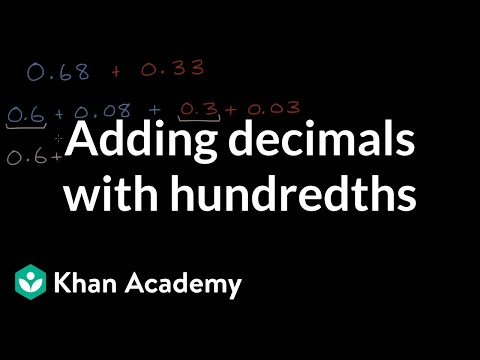Video

# Multiplying decimals: place value (Full video)

Description: Sal uses an understanding of place value to multiply 2.91 × 3.2. Created by Sal Khan. So the way I'm going to think about it is 2.91 is the same thing as 291 divided by 10. And we know that if you divide something by 100, you are going to move the decimal place two places to the left. So it makes sense that 2.91 is the same thing as 291 divided by 100.

### Other videos you might be interested in### Math Studio Talk: Instruction for 5.NBT (Full video)

#### EngageNY